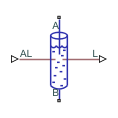# Partially Filled Pipe (IL)

Pipe with variable liquid volume in an isothermal liquid system

• Library:
• Simscape / Fluids / Isothermal Liquid / Pipes & Fittings

•## Description

The Partially Filled Pipe (IL) block models a pipe with the capacity for varying internal liquid levels. The pipe may also become completely dry during simulation.

In addition to liquid connections at ports A and B, port AL receives the inlet liquid level from connected blocks as a physical signal. If the value at AL is greater than zero, A is submerged. If the value at AL is less than or equal to zero, the port is exposed. The pipe liquid level is transmitted as a physical signal to connecting blocks at port L.

Port A is always higher than port B. If port A becomes exposed, the pipe can be filled through port B. When fluid enters the pipe through port B, the mass flow rate through port A is 0 until the pipe is fully filled, at which point ${\stackrel{˙}{m}}_{A}=-{\stackrel{˙}{m}}_{B}.$

### When to Use the Partially Filled Pipe (IL) Block

This block can be used in conjunction with the Tank (IL) block when fluid levels are expected to fall below the tank inlet. Multiple Partially Filled Pipe (IL) blocks can also be connected in series or parallel. However, because a partially filled pipe can only be filled at port B, if port A of one block in a parallel configuration becomes exposed, it may not be possible to refill this pipe if its connection at port B cannot refill the pipe.

### Pressure Loss Over the Pipe

The pressure differential over the pipe, pApB, comprises losses due to wall friction and hydrostatic pressure differences between the liquid surface height and the liquid height at port A:

`${p}_{A}-{p}_{B}=\Delta {p}_{loss}+\Delta {p}_{elev}.$`

Friction losses depend on the fluid regime in the pipe. If the flow is laminar, the friction losses are:

`$\Delta {p}_{loss}=\frac{-{\stackrel{˙}{m}}_{B}\upsilon {f}_{s}\stackrel{^}{L}}{2{D}_{h}^{2}{A}^{2}},$`

where:

• ν is the fluid kinematic viscosity.

• fS is the Laminar friction constant for Darcy friction factor.

• $\stackrel{^}{L}$ is the sum of the pipe length and its Aggregate equivalent length of local resistances, in proportion to the pipe fill level: $\stackrel{^}{L}=\left(L+{L}_{add}\right)\frac{l}{{l}_{\mathrm{max}}}.$

• l, lmax are the liquid level and the Elevation drop from port A to port B, respectively.

• Dh is the pipe hydraulic diameter. If the pipe cross-section is not circular, the hydraulic diameter is the equivalent circular diameter.

If the flow is turbulent, the friction losses are:

`$\Delta {p}_{loss}=\frac{-{\stackrel{˙}{m}}_{B}|{\stackrel{˙}{m}}_{B}|f\stackrel{^}{L}}{2\rho {D}_{h}^{2}{A}^{2}}.$`

f is the Darcy friction factor for turbulent flows, which is determined by the empirical Haaland correlation:

`$f={\left\{-1.8{\mathrm{log}}_{10}\left[\frac{6.9}{\mathrm{Re}}+{\left(\frac{\epsilon }{3.7{D}_{h}}\right)}^{1.11}\right]\right\}}^{-2},$`

where ε is the Internal surface absolute roughness. The Reynolds number is based on the mass flow rate at port B and the pipe hydraulic diameter.

The hydrostatic pressure difference is $\Delta {p}_{elev}=\rho gl.$

### Mass Flow Rate

The flow in the pipe is dictated by the internal fluid level and the conditions at port B. The pipe can be filled or drained at B if the pipe is partially empty. If the pipe is fully filled, ${\stackrel{˙}{m}}_{A}={\stackrel{˙}{m}}_{B}.$, and mass is conserved:

`${\stackrel{˙}{m}}_{A}+{\stackrel{˙}{m}}_{B}=0.$`

The mass of fluid in the pipe is determined by the relative fill level of the pipe:

`$M=\rho AL\frac{l}{{l}_{\mathrm{max}}}.$`

### Assumptions and Limitations

This block does not account for dynamic compressibility or fluid inertia, and does not model the dynamics of air (or second liquid) in the pipe.

## Ports

### Conserving

expand all

Fluid entry or exit to the pipe. Port A is always higher than port B. When port A is submerged, ${\stackrel{˙}{m}}_{A}={\stackrel{˙}{m}}_{B}.$. When port A is exposed, ${\stackrel{˙}{m}}_{A}=0.$

Fluid entry or exit to the pipe. Port A is always higher than port B. When port A is submerged, ${\stackrel{˙}{m}}_{A}={\stackrel{˙}{m}}_{B}.$ When port A is exposed, any flow into or out of port B changes the liquid level in the pipe. A dry or partially dry pipe can only be filled through port B.

### Input

expand all

Relative liquid level of the connected block in m, specified as a physical signal. If the signal at AL is positive, the pipe end is submerged. Otherwise the port is exposed.

### Output

expand all

Liquid level in the pipe in m, returned as a physical signal..

## Parameters

expand all

### Geometry

Pipe length.

Cross-sectional area of the pipe.

Hydraulic diameter used in calculations of the pipe Reynolds number. For non-circular pipes, the hydraulic diameter is the effective diameter of the fluid in the pipe. For circular pipes, the hydraulic diameter and pipe diameter are the same.

Vertical change in elevation of the pipe. Port A is always higher than port B.

Liquid level in the pipe at the beginning of the simulation.

Whether to notify if no liquid is in the pipe. Set this parameter to `Warning` if you would like to receive a warning when this occurs during simulation. Set the parameter to `Error` if you would like the simulation to stop when this occurs.

Acceleration of gravity constant.

### Friction

Length of pipe that would produce the equivalent hydraulic losses as would a pipe with bends, area changes, or other non-uniform attributes.

Pipe wall absolute roughness. This parameter is used to determine the Darcy friction factor, which contributes to pressure loss in the pipe.

Upper Reynolds number limit to laminar flow. Beyond this number, the fluid regime is transitional and approaches the turbulent regime and becomes fully turbulent at the Turbulent flow lower Reynolds number limit.

Lower Reynolds number limit for turbulent flow. Below this number, the flow regime is transitional and approaches laminar flow and becomes fully laminar at the Laminar flow upper Reynolds number limit.

Pipe friction constant for laminar flows. The laminar Darcy friction factor captures the contribution of wall friction in pressure loss calculations.

## Version History

Introduced in R2020a